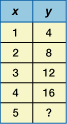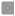## Test of Math 3.1

Grade 4 - Math - Math
1. The orchard has 206 apple trees and 385 cherry trees. About how many more cherry trees than apple trees does the orchard have?

2. What is the difference between 5,079 and 386?

3. Maureen is helping a local organization by stuffing envelopes. She must stuff 500 envelopes. She has already stuffed 321 envelopes. Which equation can be used to find the number of envelopes Maureen has left to stuff?

4. Which expression is equal to 27?

5. Use the table below to answer this question.

Which equation does the table show?6. Use the table below to answer this question.

What number is missing from the table?7. What number should go in the box to make the number sentence true?

(6 + 1) ? 3 =? 3

8. What is the value of n in the following equation?

9 + (4 ? 8) = n